JMSLTM Numerical Library 7.2.0
com.imsl.math

## Class JMath

• ```public final class JMath
extends Object```
Pure Java implementation of the standard java.lang.Math class. This Java code is based on C code in the package fdlibm, which can be obtained from www.netlib.org.
• ### Field Summary

Fields
Modifier and Type Field and Description
`static double` `E`
`static double` `PI`
• ### Method Summary

Methods
Modifier and Type Method and Description
`static double` `abs(double x)`
Returns the absolute value of a `double`.
`static float` `abs(float x)`
Returns the absolute value of a `float`.
`static int` `abs(int x)`
Returns the absolute value of an `int`.
`static long` `abs(long x)`
Returns the absolute value of a `long`.
`static double` `acos(double x)`
Returns the inverse (arc) cosine of a `double`.
`static double` `asin(double x)`
Returns the inverse (arc) sine of a `double`.
`static double` `atan(double x)`
Returns the inverse (arc) tangent of a `double`.
`static double` ```atan2(double y, double x)```
Returns the angle corresponding to a Cartesian point.
`static double` `ceil(double x)`
Returns the value of a `double` rounded toward positive infinity to an integral value.
`static double` `cos(double x)`
Returns the cosine of a `double`.
`static double` `exp(double x)`
Returns the exponential of a `double`.
`static double` `floor(double x)`
Returns the value of a `double` rounded toward negative infinity to an integral value.
`static double` ```IEEEremainder(double x, double p)```
Returns the IEEE remainder from x divided by p.
`static double` `log(double x)`
Returns the natural logarithm of a `double`.
`static double` ```max(double x, double y)```
Returns the larger of two `double`s.
`static float` ```max(float x, float y)```
Returns the larger of two `float`s.
`static int` ```max(int x, int y)```
Returns the larger of two `int`s.
`static long` ```max(long x, long y)```
Returns the larger of two `longs`.
`static double` ```min(double x, double y)```
Returns the smaller of two `double`s.
`static float` ```min(float x, float y)```
Returns the smaller of two `float`s.
`static int` ```min(int x, int y)```
Returns the smaller of two `int`s.
`static long` ```min(long x, long y)```
Returns the smaller of two `long`s.
`static double` ```pow(double x, double y)```
Returns x to the power y.
`static double` `random()`
Returns a random number from a uniform distribution.
`static double` `rint(double x)`
Returns the value of a `double` rounded toward the closest integral value.
`static long` `round(double x)`
Returns the `long` closest to a given `double`.
`static int` `round(float x)`
Returns the integer closest to a given `float`.
`static double` `sin(double x)`
Returns the sine of a `double`.
`static double` `sqrt(double x)`
Returns the square root of a `double`.
`static double` `tan(double x)`
Returns the tangent of a `double`.
• ### Methods inherited from class java.lang.Object

`clone, equals, finalize, getClass, hashCode, notify, notifyAll, toString, wait, wait, wait`
• ### Field Detail

• #### E

`public static final double E`
• #### PI

`public static final double PI`
• ### Method Detail

• #### abs

`public static double abs(double x)`
Returns the absolute value of a `double`.
Parameters:
`x` - a `double`
Returns:
a `double` representing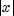.
• #### abs

`public static float abs(float x)`
Returns the absolute value of a `float`.
Parameters:
`x` - a `float`
Returns:
a `float` representing.
• #### abs

`public static int abs(int x)`
Returns the absolute value of an `int`.
Parameters:
`x` - an `int`
Returns:
an `int` representing.
• #### abs

`public static long abs(long x)`
Returns the absolute value of a `long`.
Parameters:
`x` - a `long`
Returns:
a `long` representing.
• #### acos

`public static double acos(double x)`
Returns the inverse (arc) cosine of a `double`.
Parameters:
`x` - a `double`
Returns:
a `double` representing the angle, in radians, whose cosine is x. It is in the range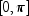.
• #### asin

`public static double asin(double x)`
Returns the inverse (arc) sine of a `double`.
Parameters:
`x` - a `double`
Returns:
a `double` representing the angle, in radians, whose sine is x. It is in the range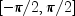.
• #### atan

`public static double atan(double x)`
Returns the inverse (arc) tangent of a `double`.
Parameters:
`x` - a `double`
Returns:
a `double` representing the angle, in radians, whose tangent is x. It is in the range.
• #### atan2

```public static double atan2(double y,
double x)```
Returns the angle corresponding to a Cartesian point.
Parameters:
`x` - a `double`, the first argument
`y` - a `double`, the second argument
Returns:
a `double` representing the angle, in radians, the the line from (0,0) to (x,y) makes with the x-axis. It is in the range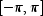.
• #### ceil

`public static double ceil(double x)`
Returns the value of a `double` rounded toward positive infinity to an integral value.
Parameters:
`x` - a `double`
Returns:
the smallest `double`, not less than x, that is an integral value
• #### cos

`public static double cos(double x)`
Returns the cosine of a `double`.
Parameters:
`x` - a `double`, assumed to be in radians
Returns:
a `double`, the cosine of x
• #### exp

`public static double exp(double x)`
Returns the exponential of a `double`. Special cases: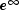is,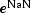is NaN;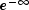is 0, and for finite argument, only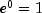is exact.
Parameters:
`x` - a `double`.
Returns:
a `double` representing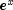.
• #### floor

`public static double floor(double x)`
Returns the value of a `double` rounded toward negative infinity to an integral value.
Parameters:
`x` - a `double`
Returns:
the smallest `double`, not greater than x, that is an integral value
• #### IEEEremainder

```public static double IEEEremainder(double x,
double p)```
Returns the IEEE remainder from x divided by p. The IEEE remainder is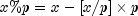as if in infinite precise arithmetic, where [x/p] is the (infinite bit) integer nearest x/p (in half way case choose the even one).
Parameters:
`x` - a `double`, the dividend
`p` - a `double`, the divisor
Returns:
a `double` representing the remainder computed according to the IEEE 754 standard.
• #### log

`public static double log(double x)`
Returns the natural logarithm of a `double`.
Parameters:
`x` - a `double`
Returns:
a `double` representing the natural (base e) logarithm of x
• #### max

```public static double max(double x,
double y)```
Returns the larger of two `double`s.
Parameters:
`x` - a `double`
`y` - a `double`
Returns:
a `double`, the larger of x and y. This function considers -0.0 to be less than 0.0.
• #### max

```public static float max(float x,
float y)```
Returns the larger of two `float`s.
Parameters:
`x` - a `float`
`y` - a `float`
Returns:
a `float`, the larger of x and y. This function considers -0.0f to be less than 0.0f.
• #### max

```public static int max(int x,
int y)```
Returns the larger of two `int`s.
Parameters:
`x` - an `int`
`y` - an `int`
Returns:
an `int`, the larger of x and y
• #### max

```public static long max(long x,
long y)```
Returns the larger of two `longs`.
Parameters:
`x` - a `long`
`y` - a `long`
Returns:
a `long`, the larger of x and y
• #### min

```public static double min(double x,
double y)```
Returns the smaller of two `double`s.
Parameters:
`x` - a `double`
`y` - a `double`
Returns:
a `double`, the smaller of x and y. This function considers -0.0 to be less than 0.0.
• #### min

```public static float min(float x,
float y)```
Returns the smaller of two `float`s.
Parameters:
`x` - a `float`
`y` - a `float`
Returns:
a `float`, the smaller of x and y. This function considers -0.0f to be less than 0.0f.
• #### min

```public static int min(int x,
int y)```
Returns the smaller of two `int`s.
Parameters:
`x` - an `int`
`y` - an `int`
Returns:
an `int` representing the smaller of x and y
• #### min

```public static long min(long x,
long y)```
Returns the smaller of two `long`s.
Parameters:
`x` - a `long`
`y` - a `long`
Returns:
a `long`, the smaller of x and y
• #### pow

```public static double pow(double x,
double y)```
Returns x to the power y.
Parameters:
`x` - a `double`, the base
`y` - a `double`, the exponent
Returns:
a `double`, x to the power y
• #### random

`public static double random()`
Returns a random number from a uniform distribution.
Returns:
a `double` representing a random number from a uniform distribution
• #### rint

`public static double rint(double x)`
Returns the value of a `double` rounded toward the closest integral value.
Parameters:
`x` - a `double`
Returns:
the `double` closest to x that is an integral value
• #### round

`public static long round(double x)`
Returns the `long` closest to a given `double`.
Parameters:
`x` - a `double`
Returns:
the `long` closest to x
• #### round

`public static int round(float x)`
Returns the integer closest to a given `float`.
Parameters:
`x` - a `float`
Returns:
the `int` closest to x
• #### sin

`public static double sin(double x)`
Returns the sine of a `double`.
Parameters:
`x` - a `double`, assumed to be in radians
Returns:
a `double`, the sine of x
• #### sqrt

`public static double sqrt(double x)`
Returns the square root of a `double`.
Parameters:
`x` - a `double`
Returns:
a `double` representing the square root of x
• #### tan

`public static double tan(double x)`
Returns the tangent of a `double`.
Parameters:
`x` - a `double`, assumed to be in radians
Returns:
a `double`, the tangent of x
JMSLTM Numerical Library 7.2.0